Sample size requirements for case-control study designs

Abstract

Background

Published formulas for case-control designs provide sample sizes required to determine that a given disease-exposure odds ratio is significantly different from one, adjusting for a potential confounder and possible interaction.

Results

The formulas are extended from one control per case to F controls per case and adjusted for a potential multi-category confounder in unmatched or matched designs. Interactive FORTRAN programs are described which compute the formulas. The effect of potential disease-exposure-confounder interaction may be explored.

Conclusions

Software is now available for computing adjusted sample sizes for case-control designs.

Background

Breslow and Day  and Smith and Day  provide asymptotic formulas for the computation of case-control sample sizes required for odds ratios, unadjusted or adjusted for a confounder  and for stratified matched designs . The notation we use is their notation. Their formulas are extended here to include more than one control per case. The formulas for stratified matched were deduced from applying the approach of Breslow and Day  (pages 305–6) to Table 7 of . Modification of the formulas for specified interactions [1, 3] is also shown. These formulas are based on the logarithm of the odds ratio, for which the normal approximation is more accurate than for the exposure difference, so these formulas are more accurate than the exposure difference formula that is given in the majority of general methods references [4, 5].

Two conversational FORTRAN programs, DAYSMITH and DESIGN, compute the formulas. They were submitted to STATLIB for non-commercial distribution a few years ago, and are obtained with an e-mail message such as "send design.exe from general" to http://statlib@lib.stat.cmu.edu. The programs produce a table of numbers of cases and controls required for a variety of specifications of Type I and Type II error, adjusted for the confounder, unadjusted, and adjusted for stratified matching, with the strata being the levels of the confounder. The two programs have different input requirements. Program DAYSMITH asks for exactly the items required for the Smith and Day formulas. Program DESIGN accepts alternative input that is converted in the program to the items required for the same formulas. The formulas used are shown in Appendix 1.

Results

The input to program DAYSMITH

The sample sizes computed are for the detection of a given disease-exposure odds ratio, that is, the sample sizes at which a certain statistical test will reject the null hypothesis that the odds ratio is one. The input items are as follows:

R E = the odds ratio to be detected (typically a minimum value),

S = 1 or 2 for one-sided or two-sided type I error,

F = the number of controls per case,

P = the control population exposure probability, and

I = an indicator to request interaction adjustment.

Roughly speaking, interaction in statistics corresponds to effect modification in epidemiology. By not selecting an interaction adjustment, we effectively assume that the disease-exposure odds ratio does not differ across confounder levels. Interaction is discussed further below.

The number of confounder levels, denoted K is asked for next. If K = 1, unadjusted sample sizes only are computed, and no other input is required. Program DESIGN is identical to this point. For most applications, no confounder adjustment is required and so the program returns unadjusted sample sizes and is finished after a 1 is entered for K. The unadjusted formula  is more accurate than the usual unadjusted formulas [4, 5], and may therefore produce different sample sizes than those.

If K > 1, one of the levels of the confounder is taken to be a reference level, and is referred to as level one. The order of the levels is otherwise immaterial. The input required next is three numbers for each of the K–1 remaining levels, p 1i , p 2i , and R Ci , i = 2,..., K, which are

p 1i = Pr(C i |E) = among the exposed population, the proportion at level i of the confounder,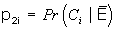= among the unexposed population, the proportion at level i of the confounder, and

R Ci = the disease-confounder odds ratio (with confounder level i versus level 1).

For the reference level, we set R c1= 1 for the formulas that follow. We compute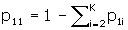and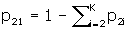.

Input for program DESIGN

Whereas DAYSMITH asks for the same input as requested in the original references , we found that alternative input made more sense for our initial applications [6, 7], so a second program was written. The input for DESIGN is the same as for DAYSMITH up to the point after which the number of levels of the confounder, K, is asked for.

Again, one of the levels of the confounder is taken to be a reference level, and is referred to as level one. The input that is required next is one number for the reference level, r i , and then three (four when interaction is included) numbers for each of the K–1 remaining levels, r i , p i , and R Ci , i = 2,...,K, which are

r i = Pr(E|C i ) = the probability of exposure at level i of the confounder,

p i = Pr(C i ) = the probability of being in level i of the confounder, and

R Ci = the odds ratio of disease and confounder level i (versus level 1).

For the reference level, we again set R Ci = 1.

From Bayes Theorem, we compute

p1i=ripi/P and

p2i=(1 – ri)pi/(1 – P).

We have one more input item than is actually required, and that is used for a check, where we can use the fact that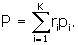What we actually do is check the sum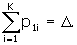The sum Δ is supposed to be equal to one. If it is not one, then we re-define and report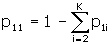and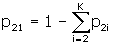,

unless they are negative. An alternative used in earlier versions was to compute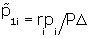and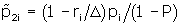and replace

p ji

with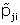for j = 1,2 and i = 1,...,K.This is equivalent to replacing

r i

with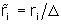,

i = 1,..., K, which is how the program used to report the change.

An example, adjusting for a confounder

The following example is one of several computations performed for a published research protocol for a study of the association of oral contraceptive (OC) use with cardiovascular risks, controlling for age group . A related protocol  has smoking as a confounder.

The numbers entered for P, r i , p i , and R Ci , i = 2,...,K, are all taken from the Saskatchewan government medical database, which includes the entire population from which a case-control sample is to be taken. In many applications, such numbers are not available from a reliable source. In that case, one may try sets of alternative minimum and maximum numbers for a range of results. The maximum sample sizes obtained from such sensitivity analyses would be the conservative recommendation.

Both programs first request R E to I. For R E , the outcome of interest is hospitalisation due to certain cardiovascular risks. The exposure is a specific OC with 10% of the market share . Since overall OC prevalence is 30%, then P = .03 for that specific OC. Using > to denote the cursor for computer entry, we type:

>2 2 3 .03 0

for R E , S, F, P and I, respectively, then press enter. We then receive the message:

Type the number of confounder levels, and <enter>. Type 1 if no confounder.

We enter 5 levels and press enter.

>5

Now type in the population exposure probability for the reference level of the confounding variable.

This will be put at level 1, so it is Pr(E|C1)

The confounder levels are five age groups, and level 1 corresponds to the youngest age group 15–21, for which we enter the prevalence for a specific OC with 10% of the market share. We type .055 and press enter.

> .055

Now type in, for each of the other 4 level(s) of the confounding variable, Pr(E|Ci), Pr(Ci), and Rc(i), separated by at least one blank or <enter>, where Pr(E|Ci) = in the population at level i of the confounder, the proportion exposed, Pr(Ci) = the probability of being at level i, and Rc(i) = odds ratio of disease and confounder level i (versus level 1).

The following numbers are entered for age groups 22–26, 27–31, 22–39 and 40+:

> .038 .24 2

> .021 .2 8

> .008 .18 8

> .004 .15 28.5

Note that Rc(5) = R C5 = 28.5, a very high value. That is to be expected if all older women are included. (For the final protocol , a cut-off was made at age 45.) When enter is pressed, we receive some confirmation of the input, and a message that the result is written to file design.out. That is, as currently written, the sample sizes and other output are not automatically shown on the screen, but are saved in "design.out" to be viewed directly there. Appendix 2 (Second attached file, app2.txt, a text file) shows the output from the preceding session, which includes a correction of the input values.

Looking at Appendix 2, we see unadjusted sample sizes, those adjusted for age in an unmatched study, and a third set of sample sizes for a matched case-control study. For our example , both unmatched and matched designs are considered. With the low value of P and the high value R C5, we see that a large difference in sample sizes required for either design may result. In most applications, however, the differences are not so dramatic.

Epidemiological literature usually gives formulas for matching which are based on the strong assumption that all sources of extraneous variation among a case and its controls are accounted for [1, 8, 9]. A third program DESIGNM was written to compute such a formula (from , p.294), but DESIGNM does not adjust for a confounding variable, and that strong assumption of implicit matching is rarely justified in case-control studies, so this program was not made freely available. Software which compute sample sizes for conditional logistic regression, such as EGRET SIZ, are alternatives to DESIGNM, which is based on Miettinen's test of the Mantel-Haenszel odds ratio for matched case-control designs. The adjustment in DAYSMITH and DESIGN is for stratified matching [2, 11, 12], where matching is by confounders. This presumes that the eventual analysis will be unconditional  and will account for the stratification. Consequently, it is not required that F controls be linked with each case, only that the total number of controls be F times the total number of cases.

Interaction

The literature [1, 3, 13, 15] discusses stratified analysis interaction adjustment only for confounders with K = 2. It is easy, however, to modify the formulas for multi-level interaction. Every occurrence of R E in the formulas (Appendix 1) is replaced by R E R Ij , where R Ij is the interaction factor corresponding to the j th level, j = 2,..., K. (For ∑', put R Ij inside the first sum.) We set R I1 = 1.

For two confounder levels, R I2, which is R I in Smith and Day's notation , is the multiplicative factor by which the odds ratio for those exposed and in level 2 of the confounder is different from the odds ratio when there is confounder-exposure-disease interaction. For R Ij , contrast is between level j and the reference level (level one).

This adjustment was made available for sensitivity analysis; specifically, to explore how much the sample size result could change if the confounder were in fact an effect modifier. Nevertheless, the adjusted formulas have been used to determine sample size in the presence of gene-environment interaction .

Discussion

The competitors to these programs are regression-based sample size programs, such as those in EGRET SIZ , which compute sample sizes required for unconditional logistic regression. The package nQuery  has an unconditional logistic regression option, but is not set up for case-control designs. These may be useful for continuous exposures, and make sense when the final analysis is intended to be such a regression, rather than a stratified analysis, such as a Mantel-Haenszel test, which our programs correspond to. We are unaware of any generally available competitor for stratified analysis.

In a series of papers on sample-size estimation to detect gene-environment interaction, which is a controversial role for sample-size formulas, comparisons have been made between regression based approaches and the stratified analysis approach [13, 15]. One solution is even to consider a case-only design . EGRET SIZ provides no guidance for interaction adjustment, but it probably could be used for that purpose.

When there is more than one confounder, we define one super-confounder, where each category corresponds to a sub-category. For example, if age, with 5 categories, and smoking, with 2 categories, are both confounders, then we define one super-confounder with 10 = 5 × 2 categories. The estimates of r i , p i , and R Ci , i = 2, ...,10, then all have to take age and smoking into account jointly. As the number of confounders and the size of K increases, regression-based sample size programs become more advantageous, since information is not required for every sub-category.

The current programs yield results for 80% and 90% power, but versions are available for alternative powers, from 60% to 95%. A new version may print to the screen, if users want that option, and ask whether sample sizes for a specific power and Type I error are required.

The programs described are for two levels of disease (case vs. control) and of exposure. For several levels of exposure or disease, measures are available which correspond to odds ratios, risk ratios and risk differences , and it is not difficult to compute sample size formulas for these. If there is some demand, software to do those calculations may be created.

The Breslow-Day-Smith formulas which we extend utilize the classical method, based on testing. A more modern approach is that based on a confidence interval for the odds ratio , which may eventually become a program option. A Bayesian approach seems most suited for the sample size problem, although some issues need to be resolved . Although not yet written, a Bayesian solution will soon be formulated for case-control designs.

References

1. 1.

Breslow NE, Day NE: Statistical Methods in Cancer Research, Vol. 2: The Design and Analysis of Cohort Studies, IARC Scientific Publications No. 82, International Agency of Research on Cancer, Lyon, France,. 1987, Sections 7.8-7.9: 305-306.

2. 2.

Smith PG, Day NE: Matching and confounding in the design and analysis of epidemiological case-control studies. Perspectives in Medical Statistics, J.F. Bithell, R. Coppi, eds. London: Academic Press,. 1987, 39-64.

3. 3.

Smith PG, Day NE: The design of case-control studies: the influence of confounding and interaction effects. International Journal of Epidemiology,. 1984, 13(3): 356-365.

4. 4.

Fleiss JL: Statistical Methods for Rates and Proportions, 2nd Edition, Wiley: New York,. 1981

5. 5.

Schlesselman JJ: Case-Control Studies: design, conduct, analysis, Oxford University Press: New York,. 1982

6. 6.

Suissa S, Hemmelgarn B, Spitzer WO, Brophy J, Collet JP, Côté R, Downey W, Edouard L, LeClerc J, Paltiel O: The Saskatchewan oral contraceptive cohort study of oral contraceptive use and cardiovascular risks. Pharmacoepidemiology and Drug Safety,. 1993, 2: 33-49.

7. 7.

Spitzer WO, Thorogood M, Heinemann L: Tri-national case-control study of oral contraceptives and health. Pharmacoepidemiology and Drug Safety,. 1993, 2: 21-31.

8. 8.

Parker RA, Bregman DJ: Sample size for individually matched case-control studies. Biometrics,. 1986, 42: 919-926.

9. 9.

Ejigou A: Power and sample size for matched case-control studies. Biometrics,. 1996, 52: 925-933.

10. 10.

EGRET. Cytel Software Corporation: Cambridge, MA,. 1997, (SIZ is a separate module)., [http://www.cytel.com]

11. 11.

Woolson RE, Bean JA, Rojas PB: Sample size for case-control studies using Cochran's statistic. Biometrics,. 1986, 42: 927-932.

12. 12.

Nam J: Sample size determination for case-control studies and the comparison of stratified and unstratified analyses. Biometrics,. 1992, 48: 389-395.

13. 13.

Hwang SJ, Beaty TH, Liang KY, Coresh J, Khoury MJ: Minimum sample size estimation to detect gene-environment interaction in case-control designs. American Journal of Epidemiology,. 1994, 140: 1029-1037.

14. 14.

Elashoff JD: nQuery Advisor relase 2.0. Statistical Solutions Ltd.: Cork, Ireland,. 1997, [http://www.statsol.ie]

15. 15.

Garcia-Closas M, Lubin JH: Power and sample size calculations in case-control studies of gene-environment interactions: comments on different approaches. American Journal of Epidemiology,. 1999, 149: 689-692.

16. 16.

Yang Q, Khoury MJ, Flanders WD: Sample size requirements in case-only designs to detect gene-environment interaction. American Journal of Epidemiology,. 1997, 146: 713-720.

17. 17.

Edwardes MD, Baltzan M: The generalization of the odds ratio, relative risk and risk difference to r × k tables. Statistics in Medicine,. 2000, 19: 1901-1914. 10.1002/1097-0258(20000730)19:14<1901::AID-SIM514>3.0.CO;2-V.

18. 18.

O'Neill RT: Sample sizes for estimation of the odds ratio in unmatched case-control studies. American Journal of Epidemiology,. 1984, 120: 145-153.

19. 19.

Joseph L, Du Berger R, Bélisle P: Bayesian and mixed Bayesian/likelihood criteria for sample size determination. Statistics in Medicine,. 1997, 16: 769-781. 10.1002/(SICI)1097-0258(19970415)16:7<769::AID-SIM495>3.0.CO;2-V.

Pre-publication history

1. The pre-publication history for this paper can be accessed here:http://0-www-biomedcentral-com.brum.beds.ac.uk/1471-2288/1/11/prepub

Acknowledgement

The author is supported by an Équipe grant from the FRSQ (Fonds de la recherche en santé du Québec). I appreciate the input of Eric Johnson, Sholom Wacholder and Jesse Berlin.

Author information

Authors

Corresponding author

Correspondence to Michael D Edwardes.

none declared

Electronic supplementary material

Appendix 1

Appendix files: - Shows the formulas utilized by DESIGN and DAYSMITH. Appendix 2 - Shows output from the DESIGN session described in the main text. (PDF 65 KB)

Appendix 1

Appendix files: - Shows the formulas utilized by DESIGN and DAYSMITH. Appendix 2 - Shows output from the DESIGN session described in the main text. (TXT 3 KB)

Rights and permissions

Reprints and Permissions

Edwardes, M.D. Sample size requirements for case-control study designs. BMC Med Res Methodol 1, 11 (2001). https://0-doi-org.brum.beds.ac.uk/10.1186/1471-2288-1-11

• Accepted:

• Published:

• DOI: https://0-doi-org.brum.beds.ac.uk/10.1186/1471-2288-1-11

Keywords

• Reference Level
• Unconditional Logistic Regression
• Input Item
• Matched Design
• Alternative Input